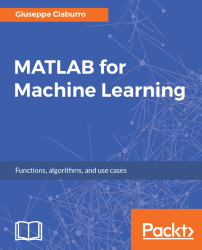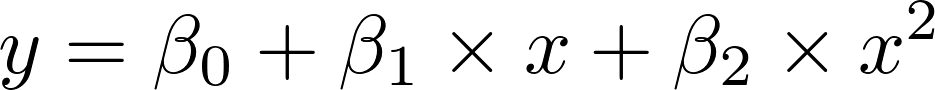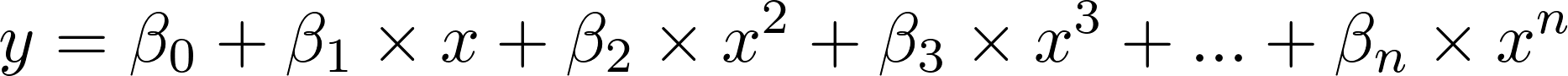•#### MATLAB for Machine Learning#### Overview of this book

MATLAB is the language of choice for many researchers and mathematics experts for machine learning. This book will help you build a foundation in machine learning using MATLAB for beginners. You’ll start by getting your system ready with t he MATLAB environment for machine learning and you’ll see how to easily interact with the Matlab workspace. We’ll then move on to data cleansing, mining and analyzing various data types in machine learning and you’ll see how to display data values on a plot. Next, you’ll get to know about the different types of regression techniques and how to apply them to your data using the MATLAB functions. You’ll understand the basic concepts of neural networks and perform data fitting, pattern recognition, and clustering analysis. Finally, you’ll explore feature selection and extraction techniques for dimensionality reduction for performance improvement. At the end of the book, you will learn to put it all together into real-world cases covering major machine learning algorithms and be comfortable in performing machine learning with MATLAB.
Title PageCreditsForewordwww.PacktPub.comCustomer FeedbackPrefaceFree Chapter
Getting Started with MATLAB Machine LearningImporting and Organizing Data in MATLABFrom Data to Knowledge DiscoveryFinding Relationships between Variables - Regression TechniquesPattern Recognition through Classification AlgorithmsIdentifying Groups of Data Using Clustering MethodsSimulation of Human Thinking - Artificial Neural NetworksImproving the Performance of the Machine Learning Model - Dimensionality ReductionMachine Learning in Practice## Polynomial regression

The linear model also includes polynomial regression, in which some predictors appear in degrees equal to or greater than 2. The model continues to be linear in the parameters. For example, a second-degree parabolic regression model looks like this:This model can easily be estimated by introducing the second-degree term in the regression model. The difference is that in polynomial regression, the equation produces a curved line, not a straight line. Polynomial regression is usually used when the relationship between the variables looks curved. A simple curve can sometimes be straightened out by transforming one or both of the variables. A more complicated curve, however, is best handled by polynomial regression.

More generally, a polynomial regression equation assumes the following form:In the next example, we will only deal with the case of a second-degree parabolic regression in MATLAB. Now, we'll show how to model data with a polynomial. We measured the temperature...